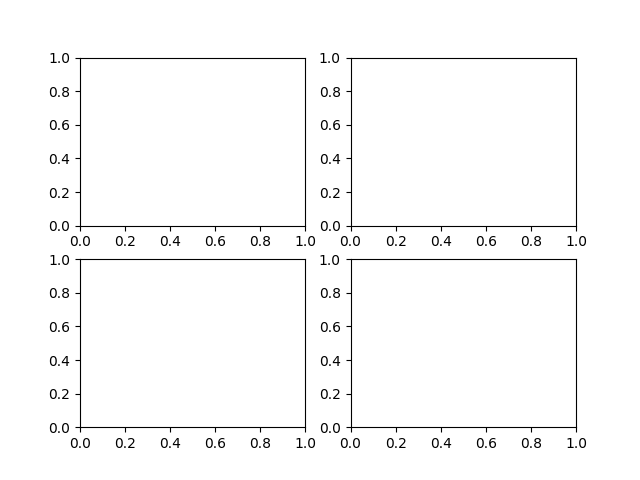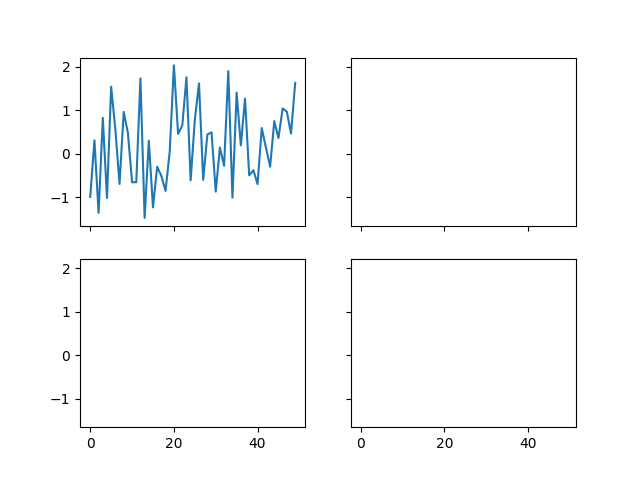# Easily creating subplots¶

In early versions of matplotlib, if you wanted to use the pythonic API and create a figure instance and from that create a grid of subplots, possibly with shared axes, it involved a fair amount of boilerplate code. e.g.

import matplotlib.pyplot as plt
import numpy as np

x = np.random.randn(50)

# old style
fig = plt.figure()Fernando Perez has provided a nice top level method to create in subplots() (note the "s" at the end) everything at once, and turn on x and y sharing for the whole bunch. You can either unpack the axes individually...

# new style method 1; unpack the axes
fig, ((ax1, ax2), (ax3, ax4)) = plt.subplots(2, 2, sharex=True, sharey=True)
ax1.plot(x)or get them back as a numrows x numcolumns object array which supports numpy indexing

# new style method 2; use an axes array
fig, axs = plt.subplots(2, 2, sharex=True, sharey=True)
axs[0, 0].plot(x)

plt.show()Keywords: matplotlib code example, codex, python plot, pyplot Gallery generated by Sphinx-Gallery